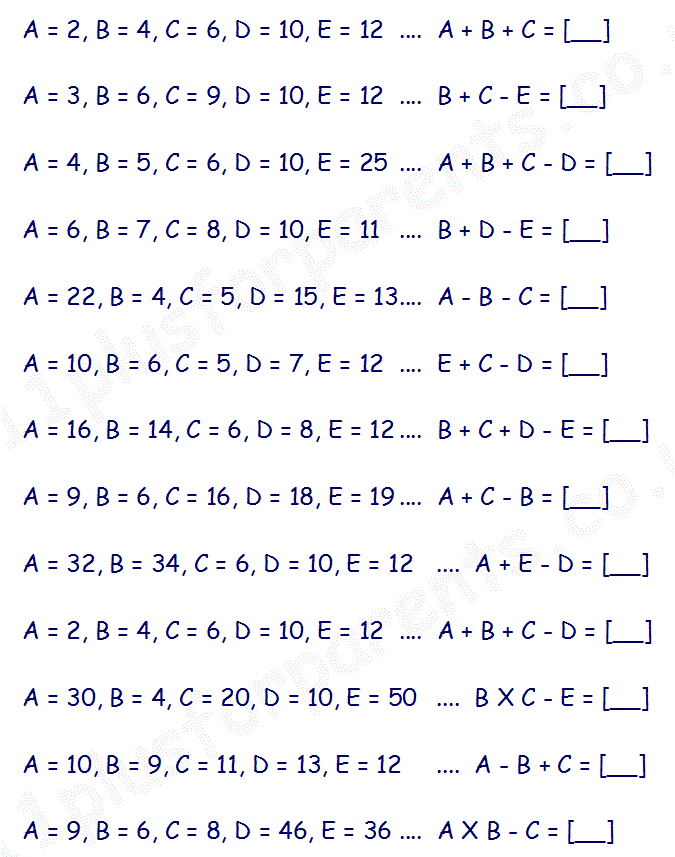# Type G - Calculating with Letters

With this type of question you are given a series of letters, each of which is assigned a number. You are also given a calculation involving the letters. You are required to work out the calculation and give the answer as a letter.

If your child has already covered Algebra in maths, they should have no problem with this.

Firstly, you need to translate the letter calculation into a number calculation. Then complete the calculation in the normal way. The numerical answer now needs to be translated back into letter form using the information at the start of the question.

Lets look at an example:

## A + B +C = [__]

rewrite the calculation in number form and complete the calculation

## 1 + 2 + 3 = 6

The answer, '6', now needs to be converted back into a letter.

## 6 = F .... The answer is F

### Verbal Reasoning Type G - 'Calculating with Letters' practice